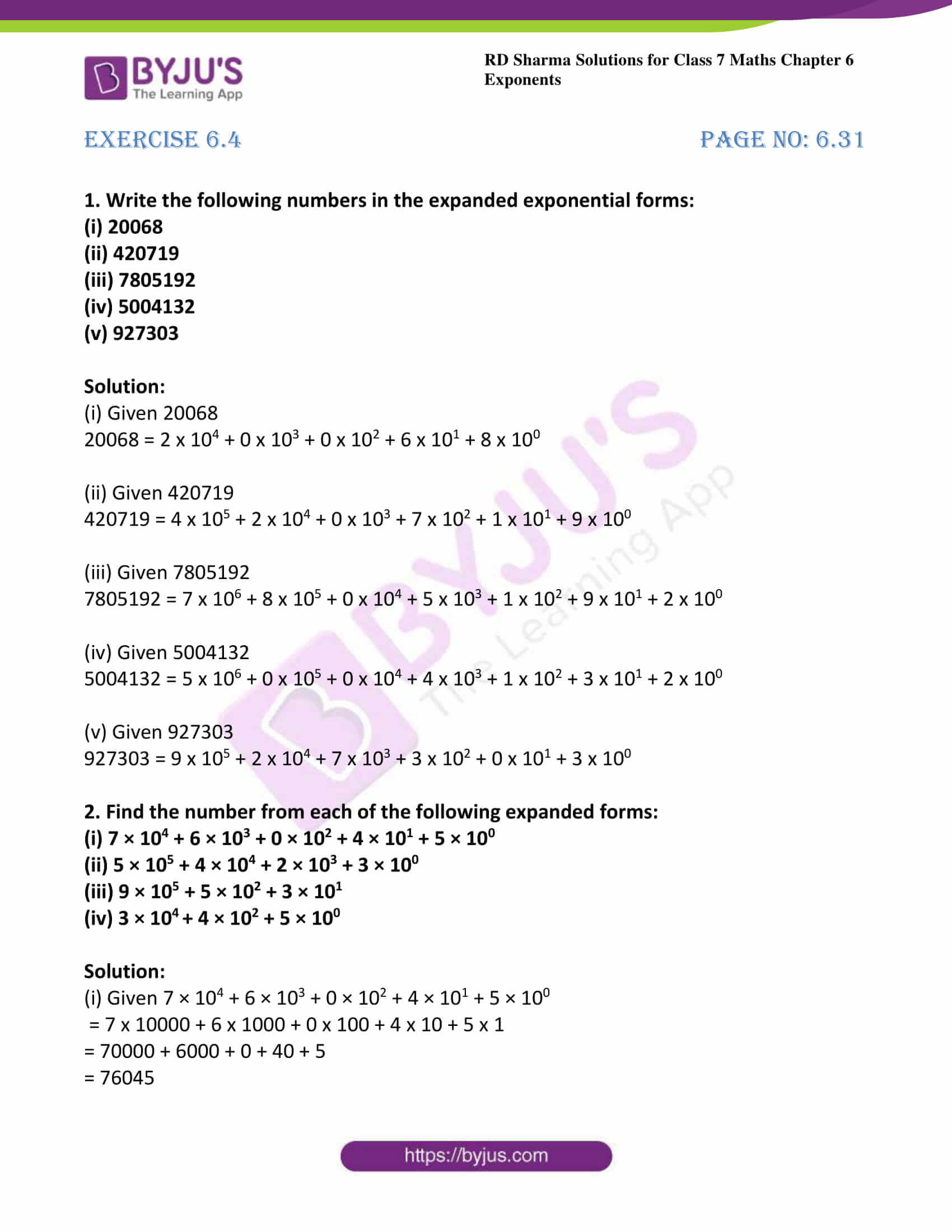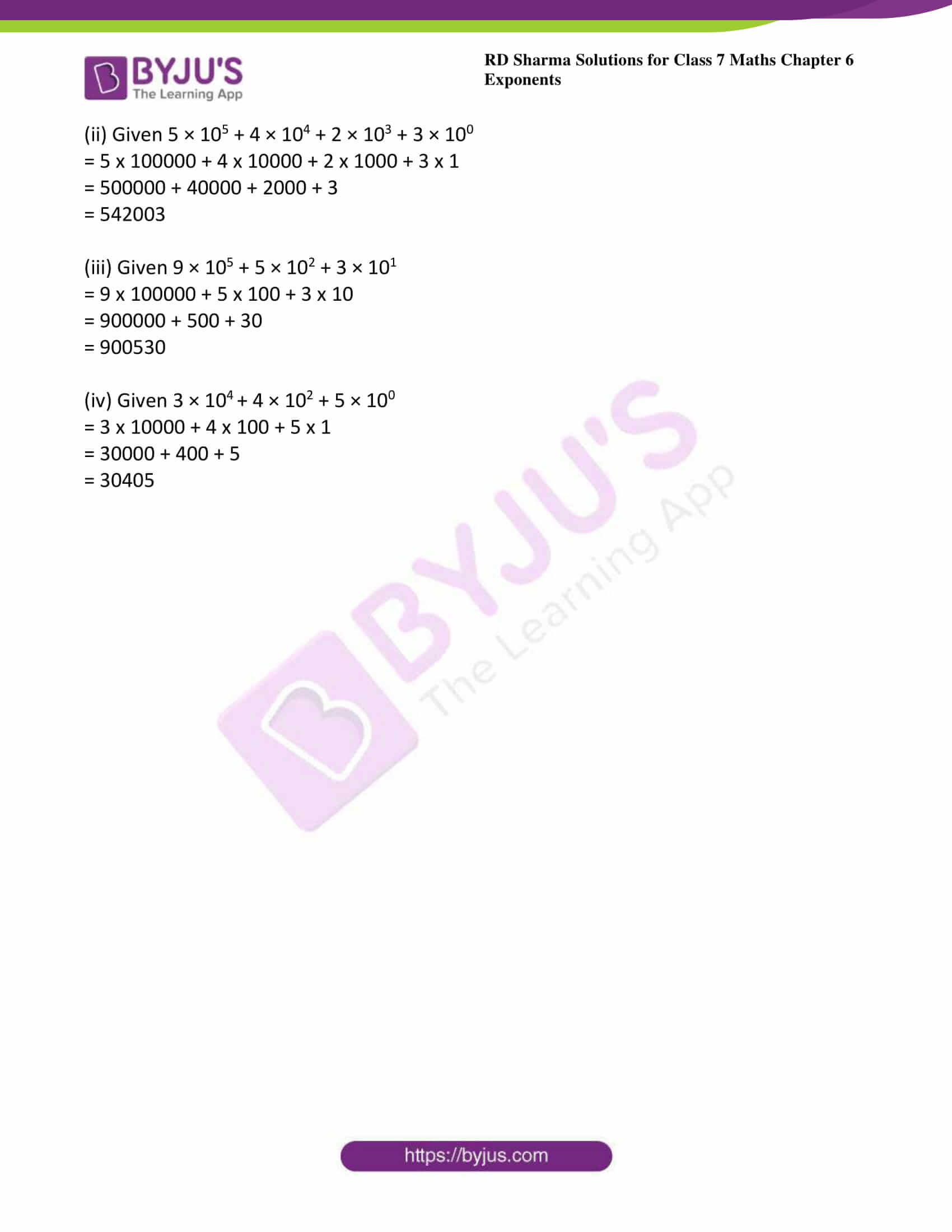# RD Sharma Solutions For Class 7 Maths Exercise 6.4 Chapter 6 Exponents

To excel in board exams, students can download the PDF of RD Sharma Solutions for Class 7 Maths Exercise 6.4 Chapter 6 Exponents which are available here. BYJU’S expert team has designed solutions efficiently, and understandably that helps students solve problems in the most efficient possible ways. By practising RD Sharma Solutions for Class 7 Maths students will thorough in Math skills. In this exercise, students will learn how to convert a given number into an expanded exponential form and vice-versa. This exercise contains two questions with many sub-questions.

## Download the PDF of RD Sharma Solutions For Class 7 Maths Chapter 6 – Exponents Exercise 6.4### Access answers to Maths RD Sharma Solutions For Class 7 Chapter 6 – Exponents Exercise 6.4

1. Write the following numbers in the expanded exponential forms:
(i) 20068
(ii) 420719
(iii) 7805192
(iv) 5004132
(v) 927303

Solution:

(i) Given 20068

20068 = 2 x 104 + 0 x 103 + 0 x 102 + 6 x 101 + 8 x 100

(ii) Given 420719

420719 = 4 x 105 + 2 x 104 + 0 x 103 + 7 x 102 + 1 x 101 + 9 x 100

(iii) Given 7805192

7805192 = 7 x 106 + 8 x 105 + 0 x 104 + 5 x 103 + 1 x 102 + 9 x 101 + 2 x 100

(iv) Given 5004132

5004132 = 5 x 106 + 0 x 105 + 0 x 104 + 4 x 103 + 1 x 102 + 3 x 101 + 2 x 100

(v) Given 927303

927303 = 9 x 105 + 2 x 104 + 7 x 103 + 3 x 102 + 0 x 101 + 3 x 100

2. Find the number from each of the following expanded forms:
(i) 7 × 104 + 6 × 103 + 0 × 102 + 4 × 101 + 5 × 100
(ii) 5 × 105 + 4 × 104 + 2 × 103 + 3 × 100
(iii) 9 × 105 + 5 × 102 + 3 × 101
(iv) 3 × 10+ 4 × 102 + 5 × 100

Solution:

(i) Given 7 × 104 + 6 × 103 + 0 × 102 + 4 × 101 + 5 × 100

= 7 x 10000 + 6 x 1000 + 0 x 100 + 4 x 10 + 5 x 1

= 70000 + 6000 + 0 + 40 + 5

= 76045

(ii) Given 5 × 105 + 4 × 104 + 2 × 103 + 3 × 100

= 5 x 100000 + 4 x 10000 + 2 x 1000 + 3 x 1

= 500000 + 40000 + 2000 + 3

= 542003

(iii) Given 9 × 105 + 5 × 102 + 3 × 101

= 9 x 100000 + 5 x 100 + 3 x 10

= 900000 + 500 + 30

= 900530

(iv) Given 3 × 10+ 4 × 102 + 5 × 100

= 3 x 10000 + 4 x 100 + 5 x 1

= 30000 + 400 + 5

= 30405